canny 订阅
canny，英语单词，主要用作形容词、名词，作形容词时译为“精明的，谨慎的；节约的”，作名词时译为“(Canny)人名；(英)坎尼”。  展开全文canny，英语单词，主要用作形容词、名词，作形容词时译为“精明的，谨慎的；节约的”，作名词时译为“(Canny)人名；(英)坎尼”。 

canny

[ˈkæni]

[ˈkæni]
canny单词用法

• ## Canny

2019-08-14 09:14:00
Canny 小记 Canny算法实现： 1.用高斯滤波器平滑图像（在调用Canny之前自己用blur平滑） 2.用一阶偏导的有限差分来计算梯度的幅值和方向． 3.对梯度幅值应用非极大值抑制 ． 4.用双阈值算法检测和连接边缘． 应用...
Canny      小记
Canny算法实现：

1.用高斯滤波器平滑图像（在调用Canny之前自己用blur平滑）
2.用一阶偏导的有限差分来计算梯度的幅值和方向．
3.对梯度幅值应用非极大值抑制 ．
4.用双阈值算法检测和连接边缘．


应用非最大抑制。这将删除不被认为是边缘的一部分的像素。因此，只有细线（候选边）将保留。
滞后：最后一步。Canny确实使用两个阈值（上限和下限）：

如果像素梯度高于上限阈值，则像素被接受为边缘
如果像素梯度值低于较低阈值，则会被拒绝。
如果像素梯度在两个阈值之间，那么只有当它连接到高于上限阈值的像素时才被接受。

阈值τ取得太低--假边缘
阈值τ取得太高--部分轮廊丢失

梯度幅值和方位角，梯度算子可自定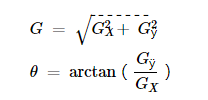此处为CSDN博主「假物者」的原创文章，遵循CC 4.0 by-sa版权协议，转载请附上原文出处链接及本声明。
原文链接：https://blog.csdn.net/qq_41248872/article/details/82889468


展开全文数字图像处理 OpenCV
• canny代码matlab ###Canny算子的硬件移植 ### 环境 使用Xilinx的FIFO和Cordic核，基于Vivado 18.2 ###简述### 包括canny的matlab代码及边缘增强模块，NMS，滞后阈值分割。
• Canny
Canny(gray-level image, output contours, low threshold, high threshold);

#include <opencv2/highgui/highgui.hpp>
#include <opencv2/imgproc/imgproc.hpp>

#include <iostream>
using namespace std;
using namespace cv;

int main()
{
Mat image_gray;
cvtColor(image,image_gray,CV_RGB2GRAY);
namedWindow("original image",WINDOW_NORMAL);
imshow("original image",image_gray);

Mat contours;
Canny(image, contours, 70, 350);
namedWindow("Canny",WINDOW_NORMAL);
imshow("Canny",contours);
waitKey(0);
}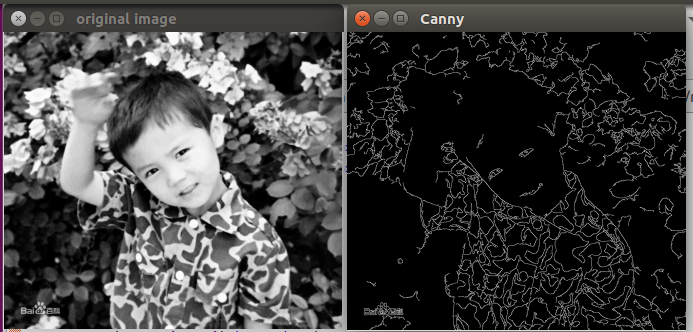来加一个threshold

#include <opencv2/highgui/highgui.hpp>
#include <opencv2/imgproc/imgproc.hpp>

#include <iostream>
using namespace std;
using namespace cv;

int main()
{
Mat image_gray;
cvtColor(image,image_gray,CV_RGB2GRAY);
namedWindow("original image",WINDOW_NORMAL);
imshow("original image",image_gray);

Mat contours;
Canny(image, contours, 70, 350);

Mat contoursInv;
threshold(contours, contoursInv, 128, 255, THRESH_BINARY_INV);
namedWindow("Canny",WINDOW_NORMAL);
imshow("Canny",contoursInv);
waitKey(0);
}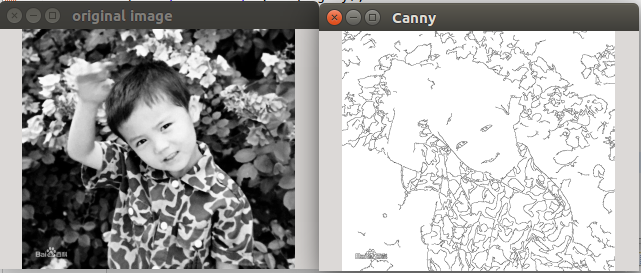展开全文• Canny算法java版 Canny边缘检测算子是John F. Canny于1986年开发出来的一个多级边缘检测算法。更为重要的是 Canny 创立了边缘检测计算理论(Computational theory of edge detection)解释这项技术如何工作。 通常...
• Zero-parameter, automatic Canny edge detection with C++ and OpenCV. This is a conversion of Adrian Rosebrock's python script to c++. Everything is contained in the include folder in auto_canny.h ...
• ## Canny算子边缘检测原理及实现

万次阅读 多人点赞 2019-06-11 12:34:09
Canny边缘检是在在1986年提出来的，到今天已经30多年过去了，但Canny算法仍然是图像边缘检测算法中最经典、先进的算法之一。 相比Sobel、Prewitt等算子，Canny算法更为优异。Sobel、Prewitt等算子有如下缺点： ...
写在前面

Canny边缘检是在在1986年提出来的，到今天已经30多年过去了，但Canny算法仍然是图像边缘检测算法中最经典、先进的算法之一。

相比Sobel、Prewitt等算子，Canny算法更为优异。Sobel、Prewitt等算子有如下缺点：

没有充分利用边缘的梯度方向。
最后得到的二值图，只是简单地利用单阈值进行处理。
而Canny算法基于这两点做了改进，提出了：

基于边缘梯度方向的非极大值抑制。
双阈值的滞后阈值处理。
原理

从表面效果上来讲，Canny算法是对Sobel、Prewitt等算子效果的进一步细化和更加准确的定位。

Canny算法基于三个基本目标：

低错误率。所有边缘都应被找到，且没有伪响应。
边缘点应该被很好地定位。已定位的边缘必须尽可能接近真实边缘。
单一的边缘点响应。这意味在仅存一个单一边缘点的位置，检测器不应指出多个像素边缘。
进而，Canny的工作本质是，从数学上表达前面的三个准则。因此Canny的步骤如下：

对输入图像进行高斯平滑，降低错误率。
计算梯度幅度和方向来估计每一点处的边缘强度与方向。
根据梯度方向，对梯度幅值进行非极大值抑制。本质上是对Sobel、Prewitt等算子结果的进一步细化。
用双阈值处理和连接边缘。
详细步骤

1、高斯平滑（略）

2、计算梯度幅度和方向

可选用的模板：soble算子、Prewitt算子、Roberts模板等等;

一般采用soble算子，OpenCV也是如此，利用soble水平和垂直算子与输入图像卷积计算dx、dy：进一步可以得到图像梯度的幅值：

为了简化计算，幅值也可以作如下近似：

角度为：

如下图表示了中心点的梯度向量、方位角以及边缘方向（任一点的边缘与梯度向量正交） ：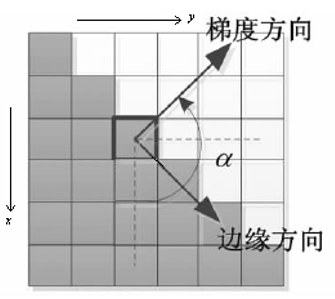3、根据角度对幅值进行非极大值抑制

划重点：是沿着梯度方向对幅值进行非极大值抑制，而非边缘方向，这里初学者容易弄混。

例如：3*3区域内，边缘可以划分为垂直、水平、45°、135°4个方向，同样，梯度反向也为四个方向（与边缘方向正交）。因此为了进行非极大值，将所有可能的方向量化为4个方向，如下图：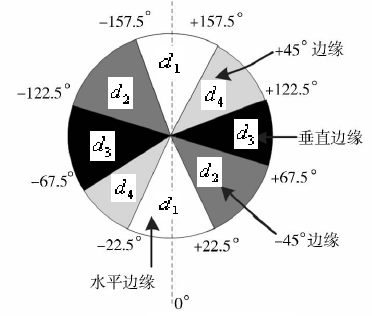量化化情况可总结为：

水平边缘--梯度方向为垂直：
135°边缘--梯度方向为45°：
垂直边缘--梯度方向为水平：
45°边缘--梯度方向为135°：
非极大值抑制即为沿着上述4种类型的梯度方向，比较3*3邻域内对应邻域值的大小：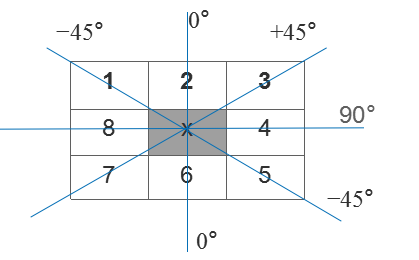在每一点上，领域中心 x 与沿着其对应的梯度方向的两个像素相比，若中心像素为最大值，则保留，否则中心置0，这样可以抑制非极大值，保留局部梯度最大的点，以得到细化的边缘。

4、用双阈值算法检测和连接边缘

选取系数TH和TL，比率为2:1或3:1。（一般取TH=0.3或0.2,TL=0.1）；
将小于低阈值的点抛弃，赋0；将大于高阈值的点立即标记（这些点为确定边缘点），赋1或255；
将小于高阈值，大于低阈值的点使用8连通区域确定（即：只有与TH像素连接时才会被接受，成为边缘点，赋 1或255）

代码实现

#include <iostream>
#include <opencv2/core.hpp>
#include <opencv2/highgui.hpp>
#include <opencv2/imgproc.hpp>
sobel算子/
//阶乘
int factorial(int n){
int fac = 1;
//0的阶乘
if (n == 0)
return fac;
for (int i = 1; i <= n; ++i){
fac *= i;
}
return fac;
}

//获得Sobel平滑算子
cv::Mat getSobelSmoooth(int wsize){
int n = wsize - 1;
cv::Mat SobelSmooothoper = cv::Mat::zeros(cv::Size(wsize, 1), CV_32FC1);
for (int k = 0; k <= n; k++){
float *pt = SobelSmooothoper.ptr<float>(0);
pt[k] = factorial(n) / (factorial(k)*factorial(n - k));
}
return SobelSmooothoper;
}

//获得Sobel差分算子
cv::Mat getSobeldiff(int wsize){
cv::Mat Sobeldiffoper = cv::Mat::zeros(cv::Size(wsize, 1), CV_32FC1);
cv::Mat SobelSmoooth = getSobelSmoooth(wsize - 1);
for (int k = 0; k < wsize; k++){
if (k == 0)
Sobeldiffoper.at<float>(0, k) = 1;
else if (k == wsize - 1)
Sobeldiffoper.at<float>(0, k) = -1;
else
Sobeldiffoper.at<float>(0, k) = SobelSmoooth.at<float>(0, k) - SobelSmoooth.at<float>(0, k - 1);
}
return Sobeldiffoper;
}

//卷积实现
void conv2D(cv::Mat& src, cv::Mat& dst, cv::Mat kernel, int ddepth, cv::Point anchor = cv::Point(-1, -1), int delta = 0, int borderType = cv::BORDER_DEFAULT){
cv::Mat  kernelFlip;
cv::flip(kernel, kernelFlip, -1);
cv::filter2D(src, dst, ddepth, kernelFlip, anchor, delta, borderType);
}

//可分离卷积———先垂直方向卷积，后水平方向卷积
void sepConv2D_Y_X(cv::Mat& src, cv::Mat& dst, cv::Mat kernel_Y, cv::Mat kernel_X, int ddepth, cv::Point anchor = cv::Point(-1, -1), int delta = 0, int borderType = cv::BORDER_DEFAULT){
cv::Mat dst_kernel_Y;
conv2D(src, dst_kernel_Y, kernel_Y, ddepth, anchor, delta, borderType); //垂直方向卷积
conv2D(dst_kernel_Y, dst, kernel_X, ddepth, anchor, delta, borderType); //水平方向卷积
}

//可分离卷积———先水平方向卷积，后垂直方向卷积
void sepConv2D_X_Y(cv::Mat& src, cv::Mat& dst, cv::Mat kernel_X, cv::Mat kernel_Y, int ddepth, cv::Point anchor = cv::Point(-1, -1), int delta = 0, int borderType = cv::BORDER_DEFAULT){
cv::Mat dst_kernel_X;
conv2D(src, dst_kernel_X, kernel_X, ddepth, anchor, delta, borderType); //水平方向卷积
conv2D(dst_kernel_X, dst, kernel_Y, ddepth, anchor, delta, borderType); //垂直方向卷积
}

//Sobel算子边缘检测
//dst_X 垂直方向
//dst_Y 水平方向
void Sobel(cv::Mat& src, cv::Mat& dst_X, cv::Mat& dst_Y, cv::Mat& dst, int wsize, int ddepth, cv::Point anchor = cv::Point(-1, -1), int delta = 0, int borderType = cv::BORDER_DEFAULT){

cv::Mat SobelSmooothoper = getSobelSmoooth(wsize); //平滑系数
cv::Mat Sobeldiffoper = getSobeldiff(wsize); //差分系数

//可分离卷积———先垂直方向平滑，后水平方向差分——得到垂直边缘
sepConv2D_Y_X(src, dst_X, SobelSmooothoper.t(), Sobeldiffoper, ddepth);

//可分离卷积———先水平方向平滑，后垂直方向差分——得到水平边缘
sepConv2D_X_Y(src, dst_Y, SobelSmooothoper, Sobeldiffoper.t(), ddepth);

//边缘强度（近似）
dst = abs(dst_X) + abs(dst_Y);
cv::convertScaleAbs(dst, dst); //求绝对值并转为无符号8位图
}

//确定一个点的坐标是否在图像内
bool checkInRang(int r,int c, int rows, int cols){
if (r >= 0 && r < rows && c >= 0 && c < cols)
return true;
else
return false;
}

//从确定边缘点出发，延长边缘
void trace(cv::Mat &edgeMag_noMaxsup, cv::Mat &edge, float TL,int r,int c,int rows,int cols){
if (edge.at<uchar>(r, c) == 0){
edge.at<uchar>(r, c) = 255;
for (int i = -1; i <= 1; ++i){
for (int j = -1; j <= 1; ++j){
float mag = edgeMag_noMaxsup.at<float>(r + i, c + j);
if (checkInRang(r + i, c + j, rows, cols) && mag >= TL)
trace(edgeMag_noMaxsup, edge, TL, r + i, c + j, rows, cols);
}
}
}
}

//Canny边缘检测
void Edge_Canny(cv::Mat &src, cv::Mat &edge, float TL, float TH, int wsize=3, bool L2graydient = false){
int rows = src.rows;
int cols = src.cols;

//高斯滤波
cv::GaussianBlur(src,src,cv::Size(5,5),0.8);
//sobel算子
cv::Mat dx, dy, sobel_dst;
Sobel(src, dx, dy, sobel_dst, wsize, CV_32FC1);

//计算梯度幅值
cv::Mat edgeMag;
if (L2graydient)
cv::magnitude(dx, dy, edgeMag); //开平方
else
edgeMag = abs(dx) + abs(dy); //绝对值之和近似

//计算梯度方向 以及 非极大值抑制
cv::Mat edgeMag_noMaxsup = cv::Mat::zeros(rows, cols, CV_32FC1);
for (int r = 1; r < rows - 1; ++r){
for (int c = 1; c < cols - 1; ++c){
float x = dx.at<float>(r, c);
float y = dy.at<float>(r, c);
float angle = std::atan2f(y, x) / CV_PI * 180; //当前位置梯度方向
float mag = edgeMag.at<float>(r, c);  //当前位置梯度幅值

//非极大值抑制
//垂直边缘--梯度方向为水平方向-3*3邻域内左右方向比较
if (abs(angle)<22.5 || abs(angle)>157.5){
float left = edgeMag.at<float>(r, c - 1);
float right = edgeMag.at<float>(r, c + 1);
if (mag >= left && mag >= right)
edgeMag_noMaxsup.at<float>(r, c) = mag;
}

//水平边缘--梯度方向为垂直方向-3*3邻域内上下方向比较
if ((angle>=67.5 && angle<=112.5 ) || (angle>=-112.5 && angle<=-67.5)){
float top = edgeMag.at<float>(r-1, c);
float down = edgeMag.at<float>(r+1, c);
if (mag >= top && mag >= down)
edgeMag_noMaxsup.at<float>(r, c) = mag;
}

//+45°边缘--梯度方向为其正交方向-3*3邻域内右上左下方向比较
if ((angle>112.5 && angle<=157.5) || (angle>-67.5 && angle<=-22.5)){
float right_top = edgeMag.at<float>(r - 1, c+1);
float left_down = edgeMag.at<float>(r + 1, c-1);
if (mag >= right_top && mag >= left_down)
edgeMag_noMaxsup.at<float>(r, c) = mag;
}

//+135°边缘--梯度方向为其正交方向-3*3邻域内右下左上方向比较
if ((angle >=22.5 && angle < 67.5) || (angle >= -157.5 && angle < -112.5)){
float left_top = edgeMag.at<float>(r - 1, c - 1);
float right_down = edgeMag.at<float>(r + 1, c + 1);
if (mag >= left_top && mag >= right_down)
edgeMag_noMaxsup.at<float>(r, c) = mag;
}
}
}

//双阈值处理及边缘连接
edge = cv::Mat::zeros(rows, cols, CV_8UC1);
for (int r = 1; r < rows - 1; ++r){
for (int c = 1; c < cols - 1; ++c){
float mag = edgeMag_noMaxsup.at<float>(r, c);
//大于高阈值，为确定边缘点
if (mag >= TH)
trace(edgeMag_noMaxsup, edge, TL, r, c, rows, cols);
else if (mag < TL)
edge.at<uchar>(r, c) = 0;
}
}
}

int main(){
cv::Mat src = cv::imread("I:\\Learning-and-Practice\\2019Change\\Image process algorithm\\Img\\lena.jpg");

if (src.empty()){
return -1;
}
if (src.channels() > 1) cv::cvtColor(src, src, CV_RGB2GRAY);
cv::Mat edge,dst;

//Canny
Edge_Canny(src, edge, 20,60);

//opencv自带Canny
cv::Canny(src, dst, 20, 80);

cv::namedWindow("src", CV_WINDOW_NORMAL);
imshow("src", src);
cv::namedWindow("My_canny", CV_WINDOW_NORMAL);
imshow("My_canny", edge);
cv::namedWindow("Opencv_canny", CV_WINDOW_NORMAL);
imshow("Opencv_canny", dst);
cv::waitKey(0);
return 0;
}

效果

与OpenCV的Canny API做了对比。

opencv的canny API:

void Canny(InputArray image, OutputArray edges, double threshold1,
double threshold2, int apertureSize=3, bool L2gradient=false )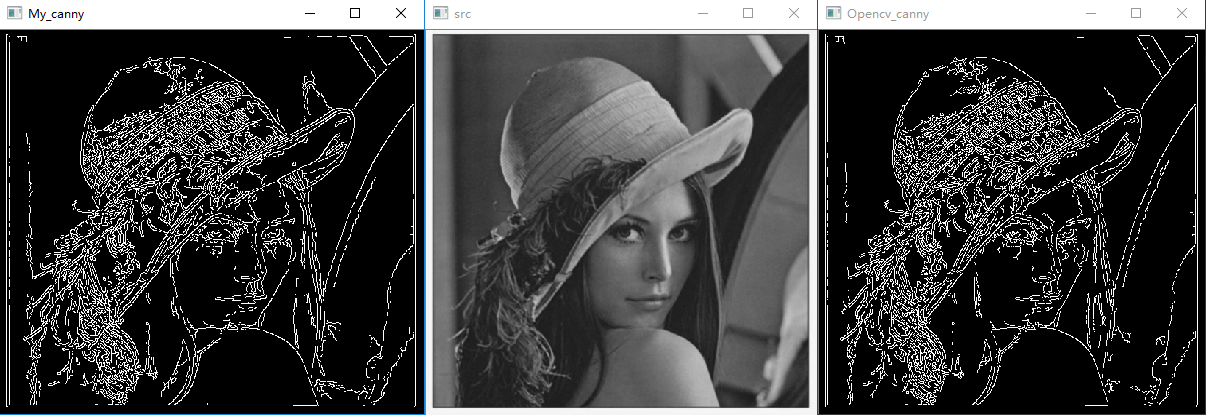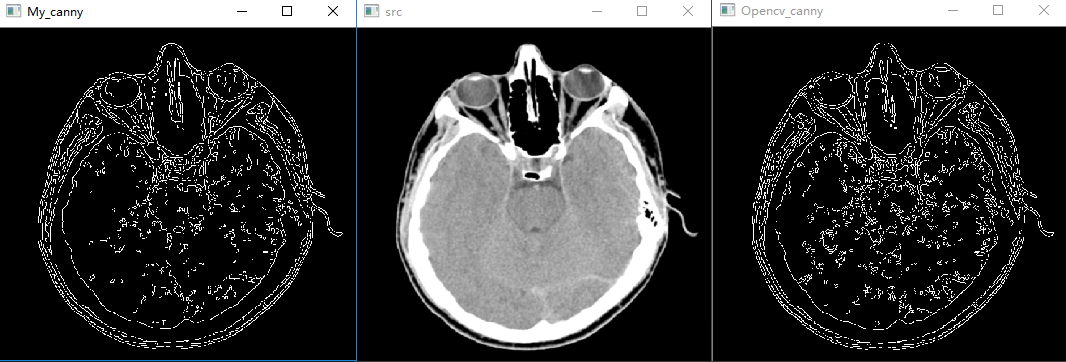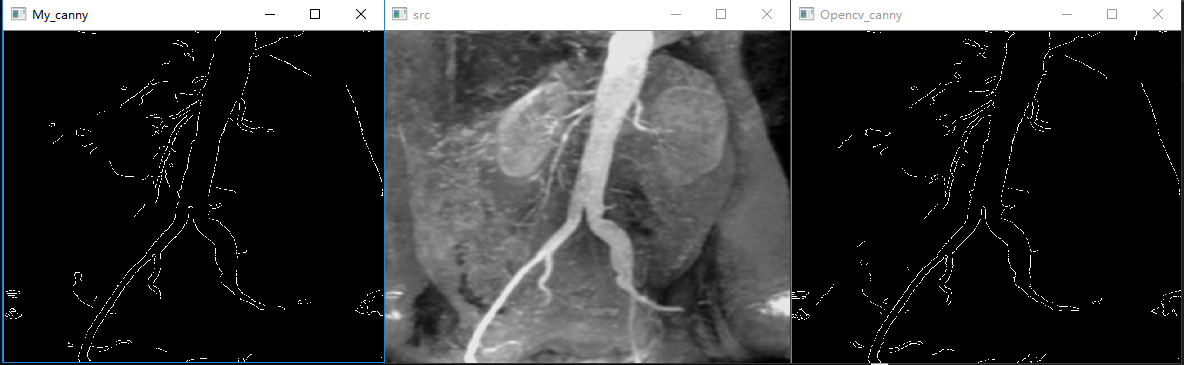一些小注意点：

atan2返回给定的 X 及 Y 坐标值的反正切值。反正切的角度值等于 X 轴与通过原点和给定坐标点 (Y坐标, X坐标) 的直线之间的夹角。结果以弧度表示并介于 -pi 到 pi 之间（不包括 -pi）。 atan2(a, b) 与 atan(a/b)稍有不同，atan2(a,b)的取值范围介于 -pi 到 pi 之间（不包括 -pi）， 而atan(a/b)的取值范围介于-pi/2到pi/2之间（不包括±pi/2)。

参考:

https://blog.csdn.net/weixin_40647819/article/list/2?

https://docs.opencv.org/3.0-last-rst/doc/tutorials/imgproc/imgtrans/canny_detector/canny_detector.html?highlight=canny

https://blog.csdn.net/liuzhuomei0911/article/details/51345591

https://blog.csdn.net/just_sort/article/details/85053157

展开全文• 使用该文档可以了解canny算子的原理及实现该程序的代码。边缘检测
• canny算子源代码，这个是canny算子在matlab中的源代码，有需要的可以一起分享。
• canny算子边缘检测-Canny.rar canny算子边缘检测，详细地代码，希望对大家有帮助 function CVHomework1 % r = imread; % I=rgb2gray;  I = imread;  BW = canny_edge;  figure,imshow  figure,imshow ...matlab
• 使用canny边缘检测算子对图像进行边缘检测，通过滑动条来改变canny的阈值，可是实时观测不同阈值下边缘检测结果。
• 该代码实现了CANNY算法的具体过程，但是与opencv的canny函数的结果比较有出入。
• ## Canny边缘检测

万次阅读 多人点赞 2018-06-02 10:08:37
Canny边缘检测是一种非常流行的边缘检测算法，是John Canny在1986年提出的。它是一个多阶段的算法，即由多个步骤构成。 1.图像降噪 2.计算图像梯度 3.非极大值抑制 4.阈值筛选 我们就事后诸葛亮，分析下这个...
Canny边缘检测是一种非常流行的边缘检测算法，是John Canny在1986年提出的。它是一个多阶段的算法，即由多个步骤构成。

1.图像降噪
2.计算图像梯度
3.非极大值抑制
4.阈值筛选

我们就事后诸葛亮，分析下这个步骤的缘由。

首先，图像降噪。我们知道梯度算子可以用于增强图像，本质上是通过增强边缘轮廓来实现的，也就是说是可以检测到边缘的。但是，它们受噪声的影响都很大。那么，我们第一步就是想到要先去除噪声，因为噪声就是灰度变化很大的地方，所以容易被识别为伪边缘。

第二步，计算图像梯度，得到可能边缘。我们在前面的关于《图像梯度》文章中有所介绍，计算图像梯度能够得到图像的边缘，因为梯度是灰度变化明显的地方，而边缘也是灰度变化明显的地方。当然这一步只能得到可能的边缘。因为灰度变化的地方可能是边缘，也可能不是边缘。这一步就有了所有可能是边缘的集合。

第三步，非极大值抑制。通常灰度变化的地方都比较集中，将局部范围内的梯度方向上，灰度变化最大的保留下来，其它的不保留，这样可以剔除掉一大部分的点。将有多个像素宽的边缘变成一个单像素宽的边缘。即“胖边缘”变成“瘦边缘”。

第四步，双阈值筛选。通过非极大值抑制后，仍然有很多的可能边缘点，进一步的设置一个双阈值，即低阈值（low），高阈值（high）。灰度变化大于high的，设置为强边缘像素，低于low的，剔除。在low和high之间的设置为弱边缘。进一步判断，如果其领域内有强边缘像素，保留，如果没有，剔除。

这样做的目的是只保留强边缘轮廓的话，有些边缘可能不闭合，需要从满足low和high之间的点进行补充，使得边缘尽可能的闭合。

我们看看效果：# 读入图像
# 图像降噪
lenna = cv2.GaussianBlur(lenna, (5, 5), 0)
# Canny边缘检测，50为低阈值low，150为高阈值high
canny = cv2.Canny(lenna, 50, 150)
cv2.imshow("canny", canny)
cv2.waitKey()

在OpenCV中，Canny函数本身应该没有将图像降噪包含在内。因此，实施Canny边缘检测时，需要在Canny函数外面执行图像降噪的过程。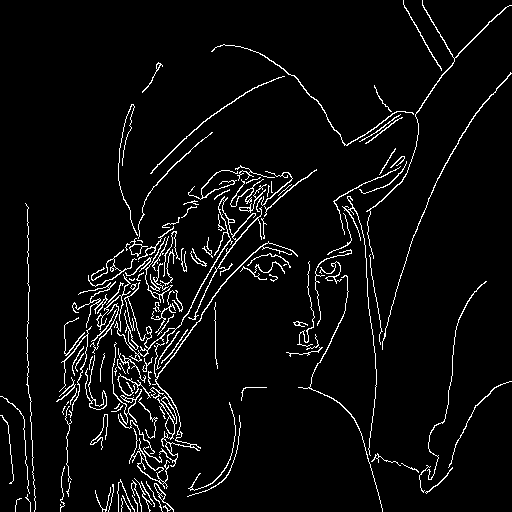调整low和high双阈值，能够得到不同的边缘效果。

canny = cv2.Canny(lenna, 100, 200)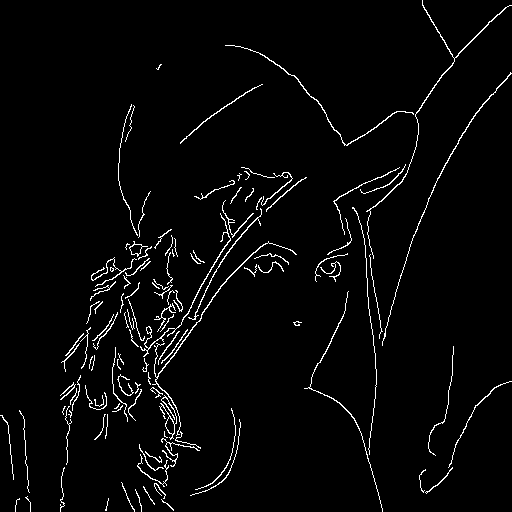再看看有噪声的情况：# 读入图像
# 图像降噪
lenna = cv2.GaussianBlur(lenna, (5, 5), 0)
# Canny边缘检测
canny = cv2.Canny(lenna, 50, 150)
cv2.imshow("canny", canny)
cv2.waitKey()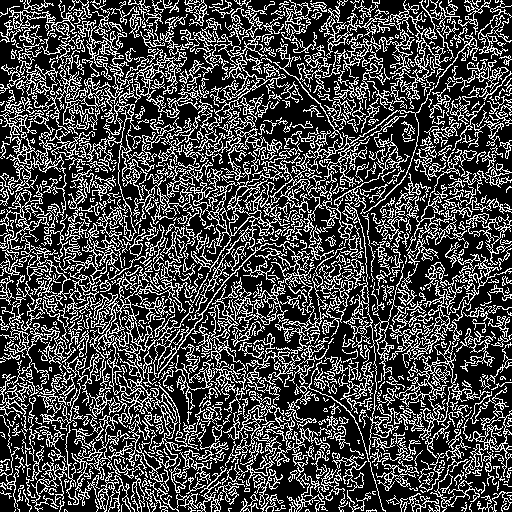效果很差啊，我们调整下高斯模糊的核大小，效果立即好了很多很多。

lenna = cv2.GaussianBlur(lenna, (9, 9), 0)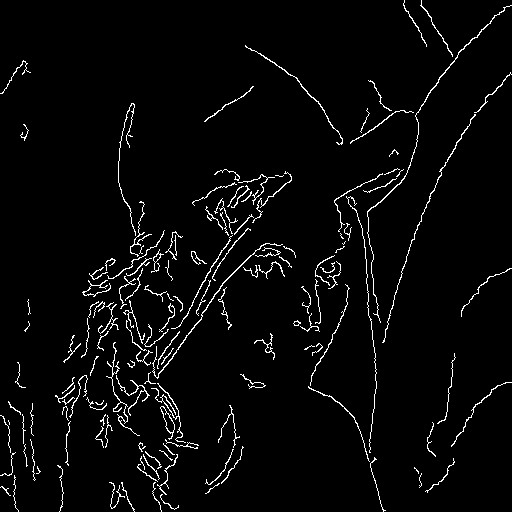所以，Canny在有噪声的情况下表现好不好，取决于前面的降噪过程，这也是为什么OpenCV将图像降噪放在Canny函数外面的原因吧，需要你自己精心调整它。
展开全文边缘检测
• 图像的边缘检测的原理是检测出图像中所有灰度值变化较大的点，而且这些点连接起来就构成了若干线条，这些线条就可以称为图像的边缘。Canny边缘检测算子是John F. Canny于 1986 年开发出来的一个多级边缘检测算法。
• 边缘检测 Canny 算法的简单实现。 步骤/算法详情： 1. 高斯滤波器系数的卷积2. 用于水平和垂直方向的 Canny 滤波器卷积3.使用atan2计算方向4. 调整到最接近的 0、45、90 和 135 度5. 非最大抑制6. 迟滞阈值 输入图像...matlab
• 主要用C++实现canny算子。没有直接调用opencv的canny算子，自己实现canny算子的函数。
• Mediafilter Canny detect
• python利用otus选定边界阈值，作为canny算子的高阈值实现，直接读取摄像头拍照，效果良好，代码简单，直接使用
•计算机视觉
• ## Canny算法

千次阅读 2018-11-30 11:04:47
Canny Canny分为5个步骤 1)、使用高斯滤波器，以平滑图像，滤除噪声。 高斯滤波器是一种平滑空间滤波器，用于模糊处理和降低噪声。我们的高斯滤波器通过以下公式得到。 我们运用该公式计算出高斯卷积核，如k越大，...数字图像处理...